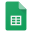# Count cells that begin with a specific text in Excel and Google Sheets

=COUNTIF(A2:A6,"feb*") // criteria within formula

=COUNTIF(A:A,C6&"*") // criteria as a cell reference

### A2:A6, A:A = ranges; C6 = criteria

Check below for a detailed explanation with pictures and how to use formulas in Excel and Google Sheets.

# Count cells that begin with a specific text in Excel

How to count cells that begin with a specific text - non-case sensitive in Excel?# COUNT CELLS THAT BEGIN WITH A SPECIFIC TEXT — EXCEL FORMULA AND EXAMPLE

1. =COUNTIF(A2:A6,"feb*") // criteria within formula

2. =COUNTIF(A:A,C6&"*") // criteria as a cell reference

• A2:A6 = data range

• * asterisk explanation

• a* = "a" at start

• *b = "b" at end

# Count cells that begin with a specific text Google Sheets

How to count cells that begin with a specific text - non-case sensitive in Google Sheets?# COUNT CELLS THAT BEGIN WITH A SPECIFIC TEXT — GOOGLE SHEETS FORMULA AND EXAMPLE

1. =COUNTIF(A2:A6,"feb*") // criteria within formula

2. =COUNTIF(A:A,C6&"*") // criteria as a cell reference

• A2:A6 = data rangeWild cards and regular expressions